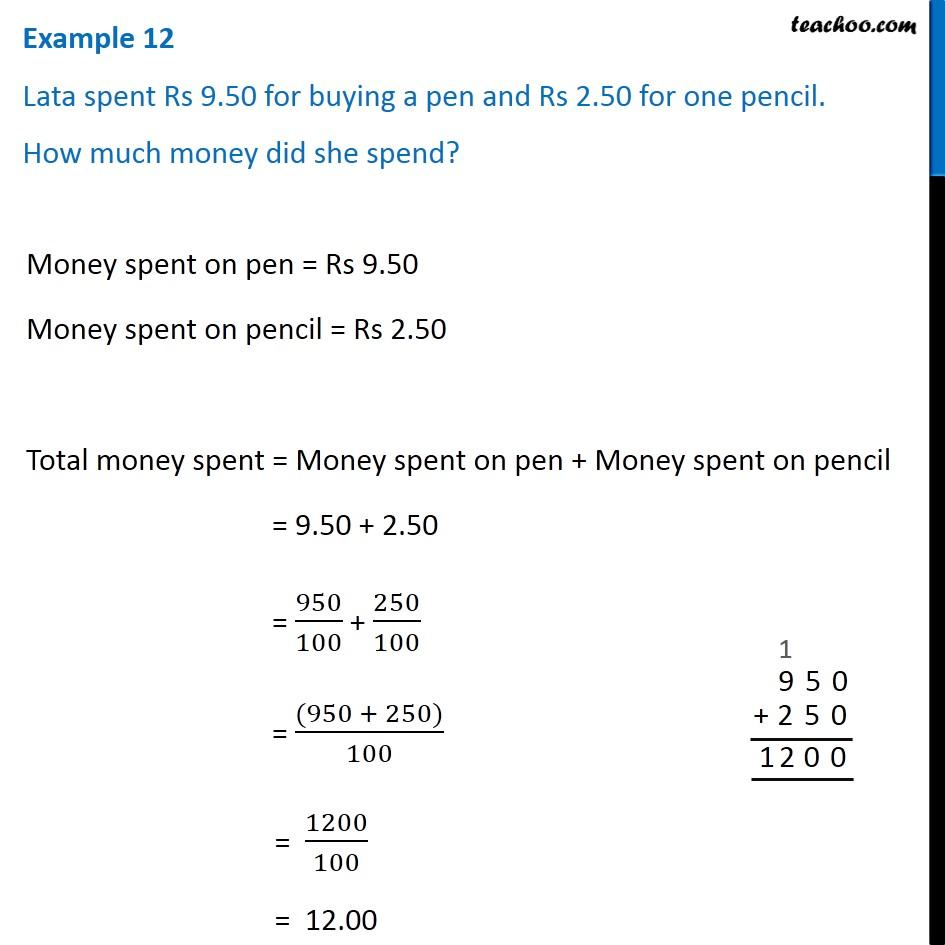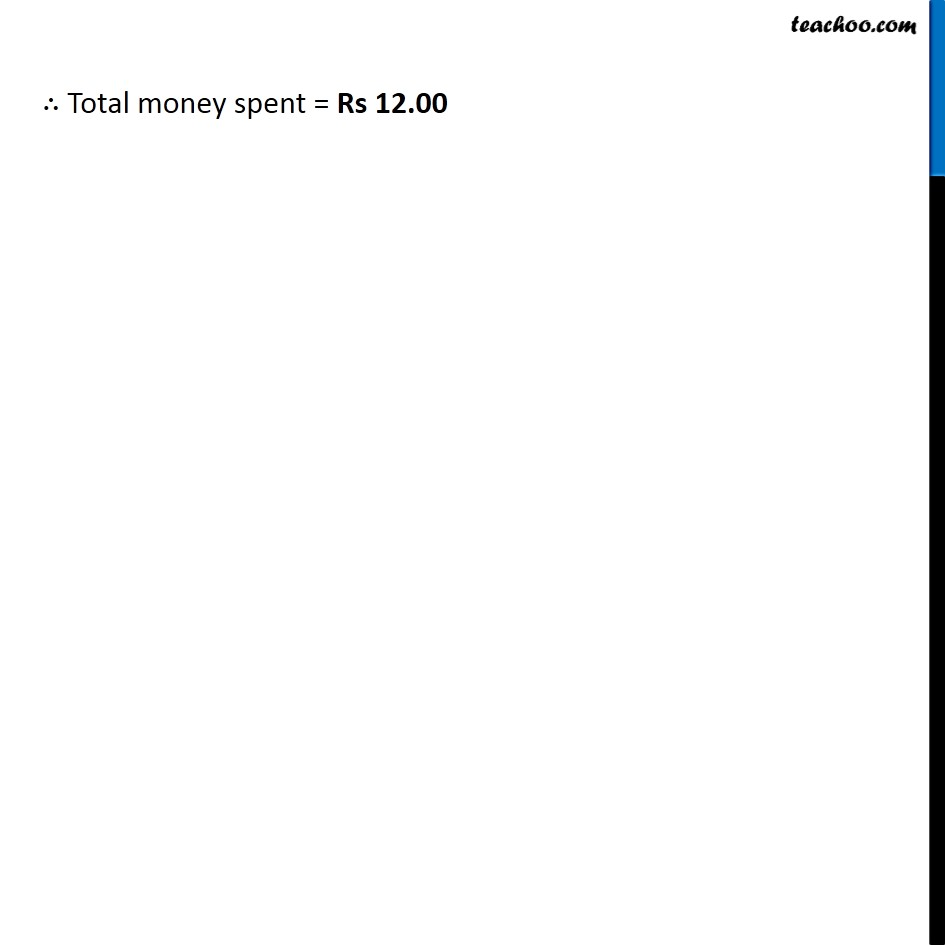Addition of decimal numbers - Statement questions

Chapter 8 Class 6 Decimals
Concept wiseLearn in your speed, with individual attention - Teachoo Maths 1-on-1 Class

### Transcript

Example 2 Lata spent Rs 9.50 for buying a pen and Rs 2.50 for one pencil. How much money did she spend?Money spent on pen = Rs 9.50 Money spent on pencil = Rs 2.50 Total money spent = Money spent on pen + Money spent on pencil = 9.50 + 2.50 = 950/100 + 250/100 = ((950 + 250))/100 = 1200/100 = 12.00 ∴ Total money spent = Rs 12.00